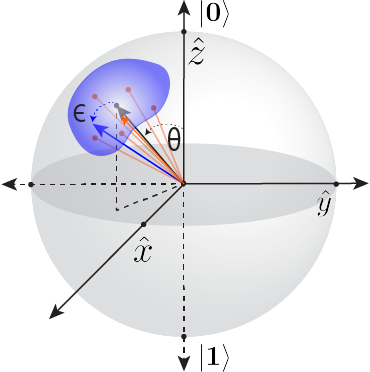In the NISQ era, it is difficult to perform quantum algorithms due to the many sources of errors that impact performance and reduce accuracy. Imprecise control pulses and classical EM crosstalk result in imperfect unitary operators, leading to coherent errors, whereas interactions with a noisy environment result in incoherent errors. Coherent errors can be particularly detrimental to algorithm performance due to the fact that they can accumulate quadratically with circuit depth. On the other hand, stochastic errors, such as bit or phase flips, only accumulate linearly with circuit depth. One method of improving algorithm performance on noisy quantum processors is through Randomize Compiling (RC) , a QCVV protocol which tailors coherent errors into stochastic Pauli errors during the implementation of a quantum circuit or algorithm. By compiling single-qubit twirling gates randomly sampled from some set into the bare sequence of an algorithm in such a way that the overall logic remains unchanged, and then combining the results of many different logically-identical circuits, the effect of coherent errors on the gates in the original circuit are averaged into stochastic noise channels that are independent of the gates themselves. Previous theoretical work  demonstrated RC improvement in simulation results for multi-qubit circuits.

The intuition behind how RC improves algorithm accuracy can be understood with the following argument, illustrated in the figure under “What we did”: Consider the final state of a single-qubit after performing a sequence of gates. The ideal final state (black vector) will lie somewhere on the surface of the Bloch sphere. The ensemble measurements of this final state will yield a probability distribution of 0’s and 1’s, which depends on where the final state of the Bloch vector is before measurement. In a circuit dominated by coherent errors, the actual final state of a bare circuit (blue vector) will be separated from the ideal state by some distance (blue surface area) due to the accumulation of over- or under-rotations during the sequence of gates. When we perform RC on a circuit, we randomize the effect that coherent errors have on the same logical circuit, so any given instance of a single RC circuit (orange points) will also be distinct from the ideal state. However, the combined distribution of all of the different RC circuits results in an average state (orange vector) that is much closer to the ideal state than is the bare state. In essence, each RC circuit represents a distinct trajectory to the same final state, and averaging together all of these trajectories mitigates the effect of coherent errors on the algorithm.